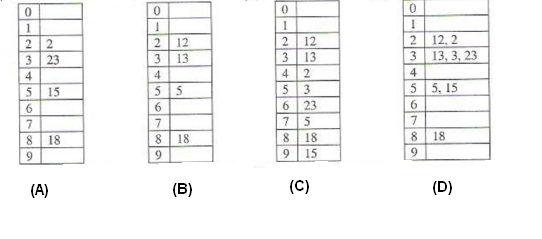# Data Structures and Algorithms | Set 19

• Last Updated : 27 Mar, 2017

Following questions have been asked in GATE CS 2009 exam.

1. Let X be a problem that belongs to the class NP. Then which one of the following is TRUE?
(A) There is no polynomial time algorithm for X.
(B) If X can be solved deterministically in polynomial time, then P = NP.
(C) If X is NP-hard, then it is NP-complete.
(D) X may be undecidable.

(A) is incorrect because set NP includes both P(Polynomial time solvable) and NP-Complete .
(B) is incorrect because X may belong to P (same reason as (A))
(C) is correct because NP-Complete set is intersection of NP and NP-Hard sets.
(D) is incorrect because all NP problems are decidable in finite set of operations.

2. What is the number of swaps required to sort n elements using selection sort, in the worst case?
(A) Θ(n)
(B) Θ(n log n)
(C) Θ(n2 )
(D) Θ(nn2 log n)

Here is Selection Sort algorithm for sorting in ascending order.

```   1. Find the minimum value in the list
2. Swap it with the value in the first position
3. Repeat the steps above for the remainder of the list (starting at
the second position and advancing each time)
```

As we can see from the algorithm, selection sort performs swap only after finding the appropriate position of the current picked element. So there are O(n) swaps performed in selection sort.
Because swaps require writing to the array, selection sort is preferable if writing to memory is significantly more expensive than reading. This is generally the case if the items are huge but the keys are small. Another example where writing times are crucial is an array stored in EEPROM or Flash. There is no other algorithm with less data movement.

References:
http://en.wikipedia.org/wiki/Selection_sort

3. The running time of an algorithm is represented by the following recurrence relation:

```    if  n <= 3  then   T(n) = n
else T(n) = T(n/3) + cn
```

Which one of the following represents the time complexity of the algorithm?
(A) Θ(n)
(B) Θ(n log n)
(C) Θ(n2)
(D) Θ(n2 log n)

```T(n) = cn + T(n/3)
= cn + cn/3 + T(n/9)
= cn + cn/3 + cn/9 + T(n/27)
Taking the sum of infinite GP series. The value of T(n) will
be less than this sum.
T(n) <= cn(1/(1-1/3))
<= 3cn/2

or we can say
cn <= T(n) <= 3cn/2
Therefore T(n) = Θ(n)
```

This can also be solved using Master Theorem for solving recurrences. The given expression lies in Case 3 of the theorem.

4. The keys 12, 18, 13, 2, 3, 23, 5 and 15 are inserted into an initially empty hash table of length 10 using open addressing with hash function h(k) = k mod 10 and linear probing. What is the resultant hash table?To get the idea of open addressing concept, you can go through below lines from Wikipedia
.
Open addressing, or closed hashing, is a method of collision resolution in hash tables. With this method a hash collision is resolved by probing, or searching through alternate locations in the array (the probe sequence) until either the target record is found, or an unused array slot is found, which indicates that there is no such key in the table. Well known probe sequences include:

linear probing in which the interval between probes is fixed--often at 1.
quadratic probing in which the interval between probes increases linearly (hence, the indices are described by a quadratic function).
double hashing in which the interval between probes is fixed for each record but is computed by another hash function.